## 1 Introduction

The notion of congruence $$n$$-permutability for varieties of universal algebras, well known in universal algebra, was extended to regular categories in : a regular category  is said to have $$n$$-permutable congruences when for any two (internal) congruences (i.e. effective equivalence relations) $$C_1$$ and $$C_2$$ on an object $$W$$ in the category, we have

\begin{aligned} (C_1,C_2)_n=(C_2,C_1)_n, \end{aligned}

where, following the notation used in , for any two relations $$S:X\rightarrow Y$$ and $$R:Y\rightarrow X$$,

\begin{aligned} (S,R)_n=\underbrace{SRSRS\dots }_{n}= \left\{ \begin{array}{l@{\quad }l}(SR)^{\frac{n}{2}}, &{} \text {when n is even,}\\ (SR)^{\frac{n-1}{2}}S, &{} \text {when n is odd.}\end{array}\right. \end{aligned}

In this paper we prove the following result, which is well known in the case when $$n=2$$ (see [4, 6]):

### Theorem 1

For a regular category $$\mathbb C$$, and a natural number $$n\geqslant 1$$, the following conditions are equivalent:

1. (i)

$$\mathbb C$$ has $$n$$-permutable congruences.

2. (ii)

$$R^\circ \leqslant R^{n-1}$$ for any internal reflexive relation $$R$$ in $$\mathbb C$$.

3. (iii)

$$R^n\leqslant R^{n-1}$$ for any internal reflexive relation $$R$$ in $$\mathbb C$$.

This result was announced for varieties in , which refers to a work of J. Hagemann in preparation. As the third author learned from L. Márki, this work was never completed to the point of publication. However, as noted in , it is not difficult to prove this result for varieties via standard arguments involving free algebras. For $$n=2$$ it gives a result due to H. Werner , which, by the way, could have inspired the discovery of the result for general $$n$$ (see the Remark in ). The authors of  seem to have missed the above result, obtaining instead a different generalization of the same result for $$n=2$$:

### Theorem 2

((ii)$$\Leftrightarrow$$(v)$$\Leftrightarrow$$(vi) of Theorem 3.5 in ) For a regular category $$\mathbb C$$, and a natural number $$n\geqslant 2$$, the following conditions are equivalent:

1. (i)

$$\mathbb C$$ has $$n$$-permutable congruences.

2. (ii)

$$(R,R^\circ )_{n-1}=(R^\circ ,R)_{n-1}$$ for any internal reflexive relation $$R$$ in $$\mathbb C$$.

3. (iii)

$$(R,R^\circ )_{n-1}$$ is transitive for any internal reflexive relation $$R$$ in $$\mathbb C$$.

## 2 Proof of Theorem 1

For a background on regular categories we refer the reader to the first few pages of , which gives a sufficiently detailed overview of regular categories from the point of view of their use for universal algebraic considerations relevant to the present paper. Apart from the definition and basic properties of a regular category, we use the calculus of relations and the language of generalised elements for regular categories, as described in .

Note that the definition of $$(S,R)_n$$ recalled in Sect. 1 makes sense for all $$n\geqslant 0$$. Indeed, for $$n=0$$ we have $$(S,R)_n=(SR)^{\frac{0}{2}}=1_Y$$ where $$1_Y$$ denotes the identity morphism $$1_Y:Y\rightarrow Y$$ regarded as a relation (the internal equality relation at $$Y$$) and for $$n=1$$ we have $$(S,R)_n=(SR)^0S=S$$. However, the property of $$n$$-permutability of congruences is “interesting” only for $$n\geqslant 2$$. Recall that 2-permutable categories are also called Mal’tsev categories  while 3-permutable categories are known as Goursat categories [3, 4].

### Lemma 1

In a regular category $$\mathbb C$$, consider a span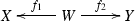and the following relations associated to it:

• the relation $$S=f_2f_1^\circ$$ defined as the composite of the opposite relation of the morphism $$f_1$$ regarded as a relation, and the morphism $$f_2$$ regarded as a relation;

• the kernel congruences $$C_1=f_1^\circ f_1$$ and $$C_2=f_2^\circ f_2$$, of $$f_1$$ and $$f_2$$ respectively.

Then for all $$n\geqslant 0$$ we have:

\begin{aligned} (C_2,C_1)_{n+1}&= f_2^\circ (S,S^\circ )_{n} f_{\frac{3}{2}+\frac{(-1)^n}{2}}\\ {f_2} (C_2,C_1)_{n+2} f_{\frac{3}{2}-\frac{(-1)^n}{2}}^\circ&= {f_2} (C_1,C_2)_{n} f_{\frac{3}{2}-\frac{(-1)^n}{2}}^\circ \\ {f_2} (C_1,C_2)_{n} f_{\frac{3}{2}-\frac{(-1)^n}{2}}^\circ&= (S,S^\circ )_{n+1} \end{aligned}

### Proof

Suppose first that $$n$$ is even. Then:

\begin{aligned} (C_2,C_1)_{n+1}&= (C_2C_1)^{\frac{n}{2}}C_2= (f_2^\circ f_2f_1^\circ f_1)^\frac{n}{2}f_2^\circ f_2\\&= f_2^\circ (f_2f_1^\circ f_1f_2^\circ )^\frac{n}{2} f_2=f_2^\circ (S S^\circ )^\frac{n}{2} f_2= f_2^\circ (S,S^\circ )_{n} f_2\\ f_2(C_2,C_1)_{n+2}f_1^\circ&= f_2(C_2C_1)^\frac{n+2}{2}f_1^\circ =f_2(f_2^\circ f_2 f_1^\circ f_1)^\frac{n+2}{2}f_1^\circ \\&= f_2f_2^\circ f_2(f_1^\circ f_1f_2^\circ f_2 )^\frac{n}{2}f_1^\circ f_1f_1^\circ =f_2(f_1^\circ f_1 f_2^\circ f_2)^{\frac{n}{2}}f_1^\circ \\&= f_2(C_1,C_2)_nf_1^\circ \\ f_2(C_1,C_2)_nf_1^\circ&= f_2(f_1^\circ f_1f_2^\circ f_2)^\frac{n}{2} f_1^\circ =(f_2 f_1^\circ f_1 f_2^\circ )^\frac{n}{2}f_2 f_1^\circ \\&= (f_2 f_1^\circ ,f_1 f_2^\circ )_{n+1}=(S,S^\circ )_{n+1} \end{aligned}

If $$n$$ is odd, then:

\begin{aligned} (C_2,C_1)_{n+1}&= (C_2C_1)^{\frac{n+1}{2}}= (f_2^\circ f_2 f_1^\circ f_1)^\frac{n-1}{2}f_2^\circ f_2f_1^\circ f_1\\&= f_2^\circ (f_2f_1^\circ f_1f_2^\circ )^\frac{n-1}{2} f_2 f_1^\circ f_1\\&= f_2^\circ (S S^\circ )^\frac{n-1}{2} S f_1 = f_2^\circ (S,S^\circ )_{n} f_1\\ f_2(C_2,C_1)_{n+2}f_2^\circ&= f_2(C_2C_1)^\frac{n+1}{2}C_2 f_2^\circ =f_2(f_2^\circ f_2 f_1^\circ f_1)^\frac{n+1}{2}f_2^\circ f_2f_2^\circ \\&= f_2(f_2^\circ f_2 f_1^\circ f_1)^\frac{n+1}{2}f_2^\circ =f_2(f_2^\circ f_2f_1^\circ f_1)^\frac{n-1}{2}f_2^\circ f_2f_1^\circ f_1f_2^\circ \\&= f_2f_2^\circ f_2(f_1^\circ f_1f_2^\circ f_2)^\frac{n-1}{2}f_1^\circ f_1f_2^\circ \\&= f_2(f_1^\circ f_1f_2^\circ f_2)^\frac{n-1}{2}f_1^\circ f_1f_2^\circ =f_2(C_1,C_2)_n f_2^\circ \\ f_2(C_1,C_2)_nf_2^\circ&= f_2(f_1^\circ f_1f_2^\circ f_2)^\frac{n-1}{2}f_1^\circ f_1f_2^\circ =(f_2f_1^\circ f_1f_2^\circ )^\frac{n-1}{2}f_2f_1^\circ f_1f_2^\circ \\&= (f_2f_1^\circ ,f_1f_2^\circ )_{n+1}=(S,S^\circ )_{n+1} \end{aligned}

$$\square$$

### Proposition 1

In the setting of Lemma 1, for any natural number $$n\geqslant 2$$ we always have $$(S,S^{\circ })_{n-1}\leqslant (S,S^{\circ })_{n+1}$$, whereas for each $$n\geqslant 1$$, the inclusion $$(S,S^{\circ })_{n+1}\leqslant (S,S^{\circ })_{n-1}$$ holds if and only if $$(C_1,C_2)_n\leqslant (C_2,C_1)_n$$.

### Proof

The fact that the inclusion $$(S,S^{\circ })_{n-1}\leqslant (S,S^{\circ })_{n+1}$$ holds for all $$n\geqslant 2$$ can be deduced from the fact that it holds for $$n=2$$, in which case it becomes

\begin{aligned} S\leqslant SS^{\circ }S. \end{aligned}

Next we show

\begin{aligned} (S,S^{\circ })_{n+1}\leqslant (S,S^{\circ })_{n-1}\,\,\Leftrightarrow \,\,(C_1,C_2)_n\leqslant (C_2,C_1)_n \end{aligned}

Suppose first $$(S,S^{\circ })_{n+1}\leqslant (S,S^{\circ })_{n-1}$$. Notice that $$(C_1,C_2)_n\leqslant (C_2,C_1)_{n+2}$$. Then, applying Lemma 1 twice we get:

\begin{aligned} (C_1,C_2)_n&\leqslant (C_2,C_1)_{n+2}=f_2^\circ (S,S^\circ )_{n+1} f_{\frac{3}{2}+\frac{(-1)^{n+1}}{2}}\\&\leqslant f_2^\circ (S,S^\circ )_{n-1} f_{\frac{3}{2}+\frac{(-1)^{n-1}}{2}}=(C_2,C_1)_n \end{aligned}

Conversely, assuming $$(C_1,C_2)_n\leqslant (C_2,C_1)_n$$ we get from Lemma 1 that for each $$n\geqslant 2$$,

\begin{aligned} (S,S^\circ )_{n+1}&= f_2(C_1,C_2)_nf_{\frac{3}{2}-\frac{(-1)^n}{2}}^\circ \leqslant f_2(C_2,C_1)_nf_{\frac{3}{2}-\frac{(-1)^n}{2}}^\circ \\&= f_2(C_1,C_2)_{n-2}f_{\frac{3}{2}-\frac{(-1)^n}{2}}^\circ =(S,S^\circ )_{n-1} \end{aligned}

For $$n=1$$ it is easy to see directly that we still get $$(S,S^{\circ })_{n+1}\leqslant (S,S^{\circ })_{n-1}$$. $$\square$$

The proposition above immediately gives the following (which in the case when $$n\geqslant 2$$ becomes (ii)$$\Leftrightarrow$$(iii) of Theorem 3.5 in ):

### Corollary 1

For any natural number $$n\geqslant 1$$, a regular category has $$n$$-permutable congruences if and only if $$(S,S^\circ )_{n+1}\leqslant (S,S^\circ )_{n-1}$$ for any internal relation $$S$$ in it.

This and the following proposition together imply Theorem 1.

### Proposition 2

In a regular category, the following conditions are equivalent for any natural number $$n\geqslant 1$$:

1. (i)

$$(S,S^\circ )_{n+1}\leqslant (S,S^\circ )_{n-1}$$ for any internal relation $$S$$.

2. (ii)

$$(S,S^\circ )_{n+1}\leqslant (S,S^\circ )_{n-1}$$ for any internal endorelation $$S$$.

3. (iii)

$$R^\circ \leqslant R^{n-1}$$ for any internal reflexive relation $$R$$.

4. (iv)

$$R^n\leqslant R^{n-1}$$ for any internal reflexive relation $$R$$.

### Proof

Let us immediately note that the implication (i)$$\Rightarrow$$(ii) is trivial. We will show (ii)$$\Rightarrow$$(iii), (iii)$$\Rightarrow$$(iv), and (iv)$$\Rightarrow$$(i).

The case when $$n=1$$ is quite straightforward. Indeed, in this case (i) states that for any relation $$S$$ from an object $$X$$ to an object $$Y$$ the composite $$SS^\circ$$ is a subrelation of the equality relation on $$Y$$, (ii) states the same for $$X=Y$$, (iii) states that the dual of any reflexive relation on an object $$A$$ coincides with the equality relation on $$A$$, while (iv) states that any reflexive relation on an object $$A$$ coincides with the equality relation on $$A$$. Thus, (iii) and (iv) are trivially equivalent. To get (ii)$$\Rightarrow$$(iv), let $$R$$ be a reflexive relation on $$A$$ and use (ii) for $$S=R$$ after noting that since $$R$$ is reflexive, we have $$R\leqslant RR^\circ$$. Finally, for (iv)$$\Rightarrow$$(i) consider an internal relation $$(s_1,s_2):S\rightarrow X\times Y$$ and note that (iv) will imply that the kernel congruence of $$s_1$$ is the equality relation on $$S$$. Hence we get the following, where the first equality comes from Lemma 1:

\begin{aligned} (S,S^\circ )_{2}=s_2 1_S s_2^\circ \leqslant s_2 s_2^\circ \leqslant 1_S=(S,S^\circ )_{0}. \end{aligned}

In the case when $$n=2$$, (i) states that any internal relation is difunctional, (ii) states that any internal endorelation is difunctional, (iii) states that any internal reflexive relation is symmetric, while (iv) states that any internal reflexive relation is transitive. The equivalence of these conditions for any finitely complete category was first obtained by A. Carboni, M.C. Pedicchio, and N. Pirovano in . Our argument for $$n\geqslant 2$$ can be seen as a generalisation of their argument, despite of the fact that the context of finitely complete categories is more general than the context of regular categories (see Remark 1). To see how to get the generalisation, it will be useful to treat the case $$n=3$$ separately.

(ii)$$\Rightarrow$$(iii) for $$n=3$$: Given an internal reflexive relation $$R\rightarrow A\times A$$, we construct a relation $$S\rightarrow R\times R$$ by taking $$S$$ to be the subobject of $$R\times R$$ whose generalised elements are given by the displays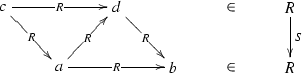To have $$R^{\circ }\leqslant R^2$$ we must show that for any two generalised elements $$a:V\rightarrow A$$ and $$b:V\rightarrow A$$ of $$A$$ such that $$bRa$$, there exists a regular epimorphism $$\gamma :U\rightarrow V$$ and a generalised element $$d:U\rightarrow A$$ such that $$a\gamma R d R b\gamma$$. Now, using the reflexivity of $$R$$ we have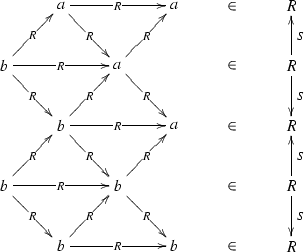(1)

The inequality $$SS^\circ SS^\circ \leqslant SS^\circ$$ applied to the above situation gives that there exists a regular epimorphism $$\gamma :U\rightarrow V$$ and there exist generalised elements $$c$$, $$d:U\rightarrow A$$ with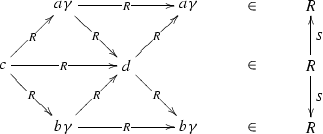(2)

from which we get $$a\gamma R d R b\gamma$$.

This argument can be exhibited by the following matrix display: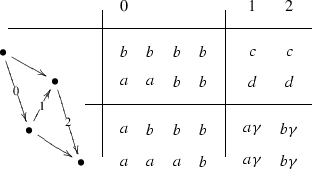Here:

• the diagram of arrows indicates how $$S$$ is constructed;

• columns represent generalised elements of $$S$$ used in the argument. The columns on the left side translate the display (1). In particular, the first column indicates that $$(b,a)S(a,a)$$, i.e. that $$(a,a)S^\circ (b,a)$$, which comes from the top part of the relations displayed in (1). The label $$0$$ of the first column of variables indicates which pair of variables from that column was assumed to belong to $$R$$ in the beginning of the argument—it is the pair that corresponds to the arrow with the same label $$0$$;

• in a similar way, the columns on the right side sketch the display (2), with the numerical labels giving the required conclusion of the argument.

(iii)$$\Rightarrow$$(iv) for $$n=3$$: In this case, the required argument can be summarised using a similar matrix display: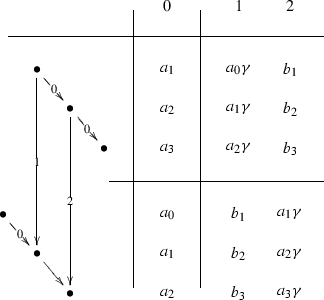In detail, given an internal reflexive relation $$R\rightarrow A\times A$$, construct another one $$S\rightarrow X\times X$$ as follows: generalised elements of $$X$$ are triples $$(a,b,c)$$ of generalised elements of $$A$$ such that $$aRbRc$$, and $$S$$ is defined by

\begin{aligned} (a,b,c)S(a',b',c') \,\,\Leftrightarrow \,\, a Rb'\, and \,b Rc'. \end{aligned}

It is easy to see that $$S$$ is a reflexive relation. Now, using $$S^{\circ }\leqslant S S$$ we want to show that $$R R R\leqslant R R$$. Assume $$a_0Ra_1Ra_2Ra_3$$ where $$a_0,a_1,a_2,a_3$$ are generalised elements of $$A$$. Then, $$(a_1,a_2,a_3)S(a_0,a_1,a_2)$$ which gives

\begin{aligned} (a_0\gamma ,a_1\gamma ,a_2\gamma )S(b_1,b_2,b_3)S(a_1\gamma ,a_2\gamma ,a_3\gamma ) \end{aligned}

for some regular epimorphism $$\gamma$$ and generalised elements $$b_1,b_2,b_3$$ of $$A$$ (having the same domain as $$\gamma$$). By the definition of $$S$$ we get $$a_0\gamma Rb_2Ra_3\gamma$$.

(iv)$$\Rightarrow$$(i) for $$n=3$$: This proof is sketched by the following matrix display: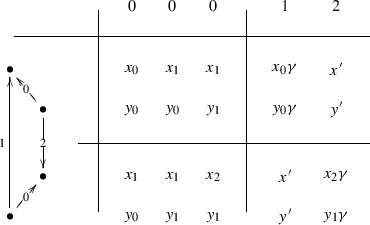This proves Proposition 2 for $$n=3$$. The proof for a general $$n\geqslant 2$$ is sketched by the matrices displayed in Figs. 1, 2, 3, 4 and 5 below.

$$\square$$

## 3 Final remarks

While working on  with N. Martins-Ferreira, the third author noticed that Theorem 1 above, announced for varieties in , did not appear in . In  the varietal proof was sketched, essentially based on the syntactical characterisation of $$n$$-permutability obtained in . In  the second and the third authors looked at extending this proof to regular categories with sums, based on the technique of approximate operations introduced by the first author and D. Bourn in . The problem of obtaining Theorem 1 for regular categories free of sums remained open. We then looked at transforming the varietal proof into a categorical one, based on an insight suggested by the algorithm for translating linear Mal’tsev conditions into categorical conditions described by the first author in .

In the literature, when one speaks of $$n$$-permutability one usually assumes $$n\geqslant 2$$. It is easy to see that in the case when $$n=1$$, this property becomes equivalent to the regular category being a preorder, while for $$n=0$$ it holds trivially. The cases when $$n=2$$ and $$n=3$$ are the best known ones. Regular categories with $$2$$-permutable congruences were called Mal’tsev categories in , following J.D.H. Smith who called varieties with $$2$$-permutable congruences Mal’tsev varieties in , after A.I. Mal’tsev who studied them in . Since , the term “Mal’tsev category” refers to a finitely complete category where every internal reflexive relation is an equivalence relation (or equivalently, where every internal relation is difunctional). Thus, in modern terminology, regular categories with $$2$$-permutable congruences are the same as regular Mal’tsev categories. Regular categories with $$3$$-permutable congruences are known as Goursat categories since  and .

We would like to point out that there are several obvious ways in which one could extend the results we obtain in this paper, to more general axiomatic contexts than that of regular categories. For example, the proof of Proposition 2 can be easily adapted to prove the following:

### Proposition 3

For any full subcategory $$\mathbb C$$ of a regular category $$\mathbb X$$, closed under finite limits in $$\mathbb X$$, the following conditions are equivalent for any natural number $$n\geqslant 1$$:

1. (i)

$$(S,S^\circ )_{n+1}\leqslant (S,S^\circ )_{n-1}$$ for any internal relation $$S\rightarrow X\times Y$$ in $$\mathbb X$$ where $$S,X,Y\in \mathbb C$$.

2. (ii)

$$(S,S^\circ )_{n+1}\leqslant (S,S^\circ )_{n-1}$$ for any internal relation $$S$$ as above with $$X=Y$$.

3. (iii)

$$R^\circ \leqslant R^{n-1}$$ for any internal reflexive relation $$R\rightarrow A\times A$$ in $$\mathbb X$$ where $$R,A\in \mathbb C$$.

4. (iv)

$$R^n\leqslant R^{n-1}$$ for any internal reflexive relation $$R$$ as above.

### Remark 1

In the above proposition, setting $$n=2$$ and $$\mathbb X =\mathbf Set ^\mathbb{C ^\mathrm op }$$, and regarding $$\mathbb C$$ as a full subcategory of $$\mathbb X$$ via the Yoneda embedding, we get Proposition 1.2 in , which establishes the equivalence between difunctionality of internal relations, and symmetry/transitivity of internal reflexive relations in any finitely complete category.

A natural question to ask is whether such generalisations would allow to obtain a characterisation of $$n$$-permutability in interesting classes of universal algebras other than (quasi)varieties. In fact, already noting that in Proposition 3 it is sufficient to require that $$\mathbb C$$ is closed in $$\mathbb X$$ under powers and equalisers, gives some new examples. Indeed, in any variety $$\mathcal V$$ take any family $$(\mathcal V _i)_{i\in I}$$ of subvarieties; then, their join $$\mathcal U =\bigvee _{i\in I}\mathcal V _i$$ gives a class of algebras in $$\mathcal V$$ which is closed under all powers and subalgebras, and this would give that the conditions of Proposition 3 for $$\mathbb C =\mathcal U$$ and $$\mathbb X =\mathcal V$$ are equivalent. In fact, they hold if and only if each $$\mathcal V _i$$ has $$n$$-permutable congruences. There are also examples which are not of this type; for instance, consider groups among all monoids... in each case, we could also restrict to, say, finite universal algebras.

Finally, we would like to note that the arguments we used to prove Proposition 2 have many variations which could allow to obtain other similar characterisations of $$n$$-permutability. For instance, the matrix display in Fig. 3, which can be used to prove 2(iii)$$\Rightarrow$$ 2(iv) for $$n\geqslant 2$$, can be used similarly to prove that 2(iii) implies the following condition:

($$*$$) For any internal relation $$R$$ on an object $$X$$ in $$\mathbb C$$,

\begin{aligned} R\leqslant (1_X\wedge R)R\Rightarrow R^n\leqslant R^{n-1}. \end{aligned}

Notice that 2(iii)$$\Rightarrow$$($$*$$) still holds for $$n=1$$, since $$1$$-permutability, as mentioned earlier, gives that $$\mathbb C$$ is a preorder, while in any preorder any relation $$R$$ on $$X$$ is contained in the equality relation $$1_X$$. Next, ($$*$$) implies 2(iv) since when $$R$$ is reflexive, $$R=(1_X\wedge R)R$$. So ($$*$$) also characterises $$n$$-permutability. One can also get this way conditions involving more than one relation. Here is one example:

($$**$$) For any two internal relations $$S,T$$ in $$\mathbb C$$,

\begin{aligned} S\leqslant T\Rightarrow (S^\circ T)^{n}\leqslant (S^\circ T)^{n-1}. \end{aligned}

This obviously implies 2(iv) since, if we take $$T$$ above to be a relation $$T=R$$ on $$X$$ and $$S=1_X$$, then we get exactly the statement in 2(iv). At the same time, ($$**$$) can be deduced from ($$*$$) by setting $$R=S^\circ T$$, since then $$S\leqslant T$$ implies $$R\leqslant (1_X\wedge R)R$$. So ($$**$$) also characterises $$n$$-permutability.